# CubesMany things, like 6-sided dice, sugar cubes and Rubik's cubes are shaped like cubes. They are chosen for the most commonly used dice because every face has the same shape and size.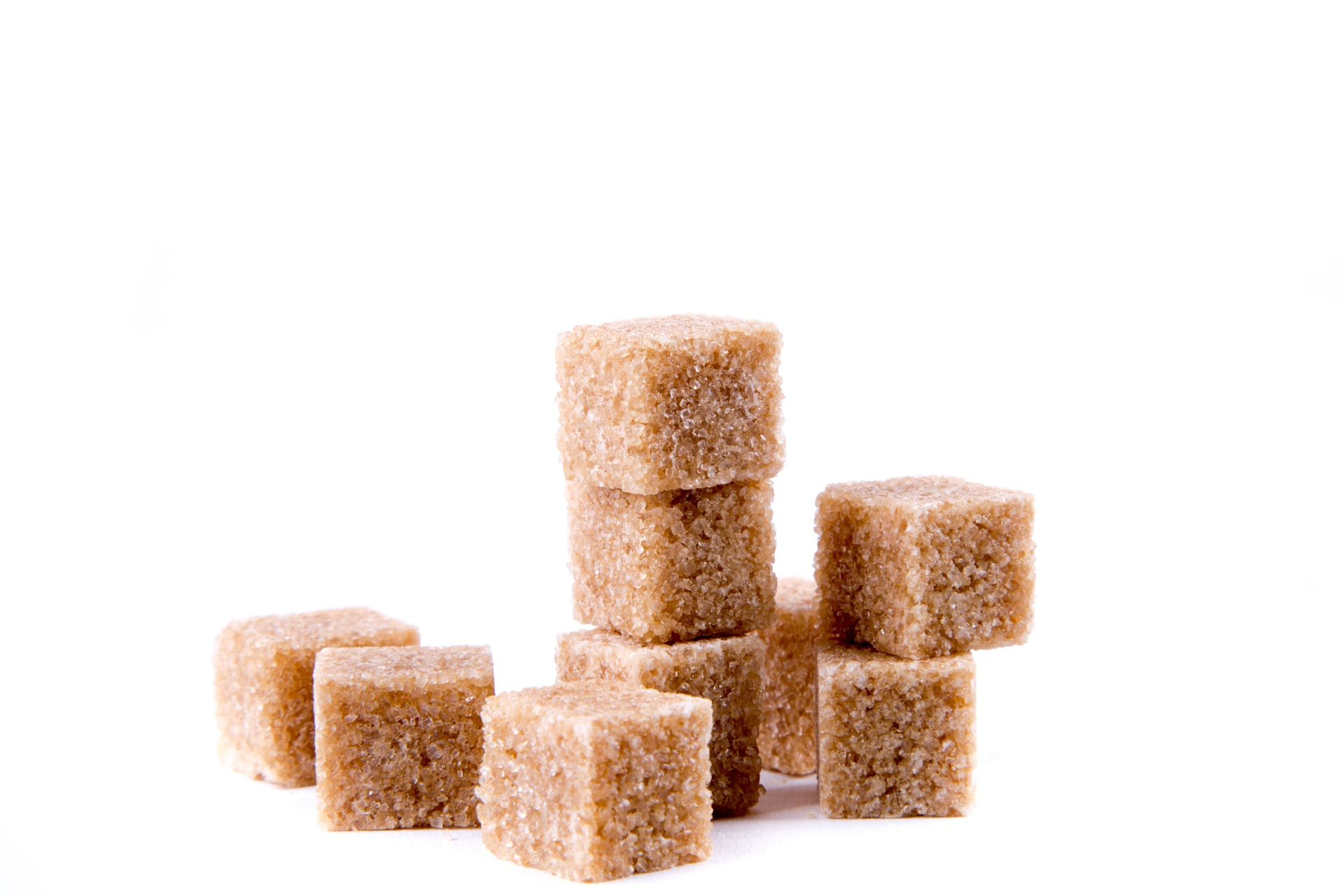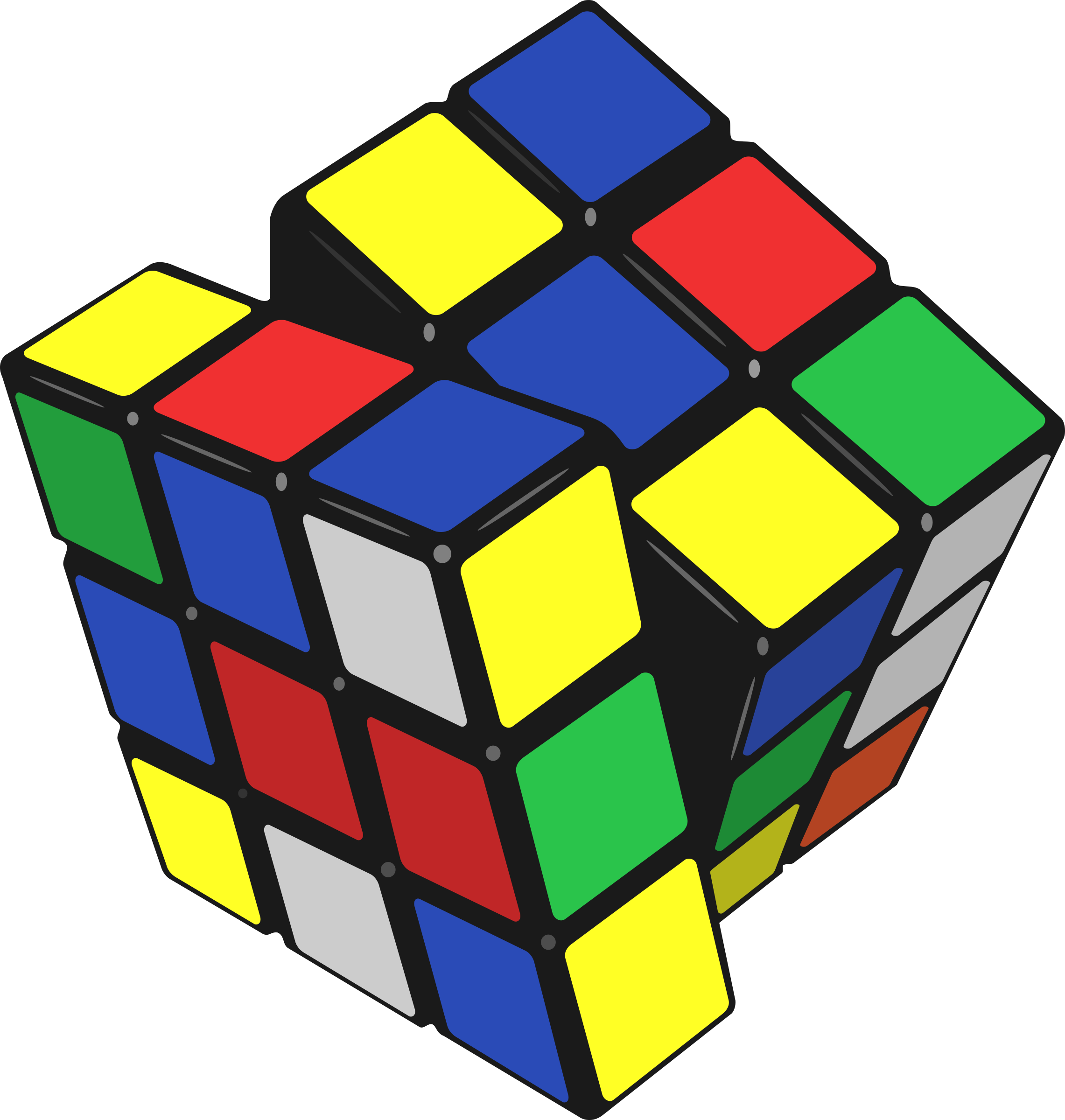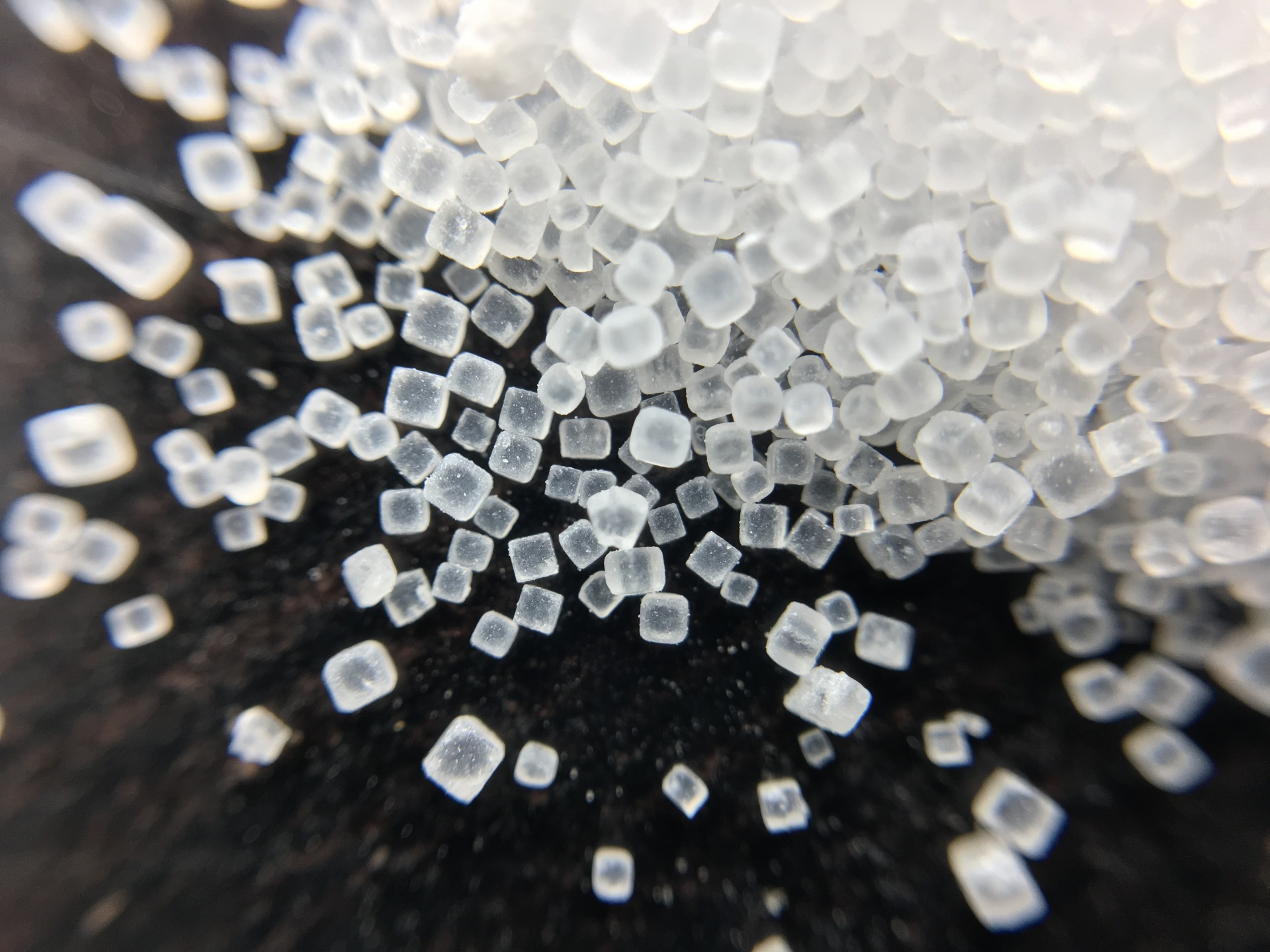Cubes also occur naturally as the crystal form for many minerals because the atoms join together as the corners of a cube. For example, salt (left) and iron pyrite (right) naturally form crystals in the shape of cubes. We call objects that are shaped like cones cubic. So, what exactly is a cube, and what are its properties?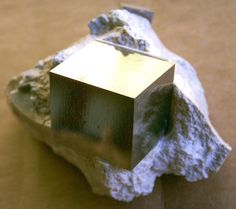## The Properties of a Cube

Cubes are solids with a six flat faces. Each flat face has the same size and shape: it has four edges, four right-angled corners, and is shaped like a square.

A cube has 12 edges and 8 vertices (corner points). Three edges meet at each vertex.

Another name for a cube is a hexahedron: cubes are polyhedra (solids with flat faces) with six (hexa-) sides.

Cubes are one of the $5$ Platonic solids.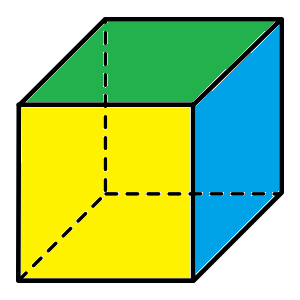## Building a Cube

You can make a cube yourself by printing the following net (template), cutting it out along the solid lines, folding it along the dotted lines and taping the edges together.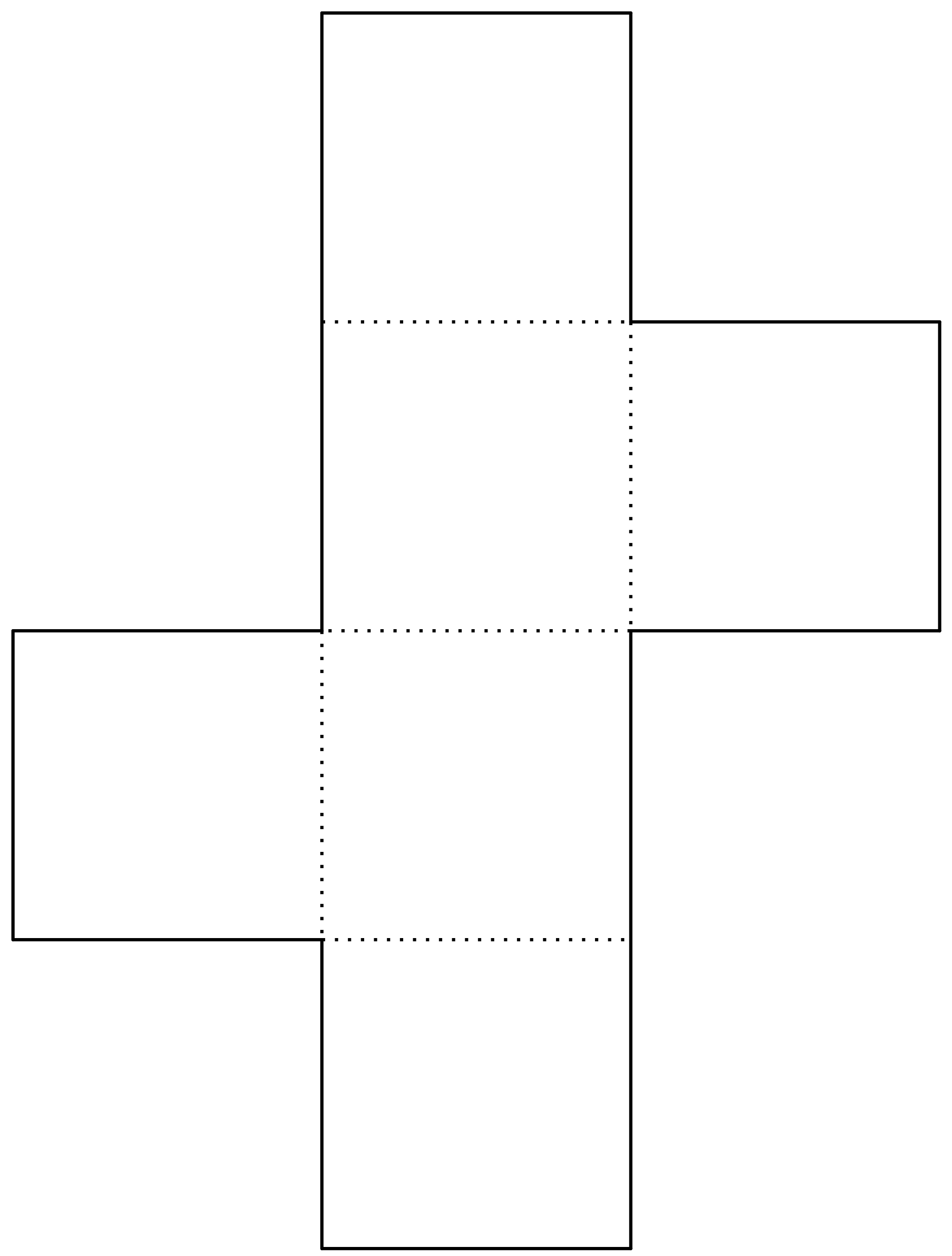## Finding the Surface Area and Volume of a Cube

Because a cube is a regular polyhedron, every edge of the cube has the same length.

### Finding the Surface Area of a Cube

The cube has $6$ sides, each of which is shaped like a square. As the area of a square is equal to the square of its side length,

$\text{Surface Area} = 6 \times (\text{Edge Length})^2$

For example, the surface area of a cube with edge length $3$ cm is

\begin{align*} \text{Surface Area} &= 6 \times (\text{Edge Length})^2\\ &= 6 \times (3)^2\\ &= 6 \times 9\\ &= 54 \text{ cm}^2 \end{align*}

### Finding the Volume of a Cube

The cube is a rectangular prism in which all three of the length, width and height are equal. So, its volume is given by

$\text{Volume} = (\text{Edge Length})^3$

For example, the volume of a cube with edge length $3$ cm is

\begin{align*} \text{Volume} &= (\text{Edge Length})^3\\ &= (3)^3\\ &= 27 \text{ cm}^3 \end{align*}

### Description

There are several lessons related to 3D geometry such as

1. Euler's formula
2. Vertices, Edges and Faces
3. Volumes of 3D shapes
4. etc

Even though we've titled this lesson series to be more inclined for Year 10 or higher students, however, these lessons can be read and utilized by lower grades students.

### Prerequisites

Understanding of 3D shapes

### Audience

Year 10 or higher, but suitable for Year 8+ students

### Learning Objectives

Get to know 3D Geometry

Author: Subject Coach
You must be logged in as Student to ask a Question.